• +91 9971497814
• info@interviewmaterial.com

# Chapter 4 Linear Equations in Two Variables Ex-4.3 Interview Questions Answers

### Related Subjects

Question 1 :

Draw the graph of each of the following linear equations in twovariables:

(i)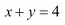(ii)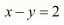(iii) y =3(iv) 3 = 2x + y

Answer 1 : (i)It can be observed that x = 0, y = 4and x = 4, y = 0 are solutions of the aboveequation. Therefore, the solution table is as follows.

 x 0 4 y 4 0

The graph of this equation is constructed as follows.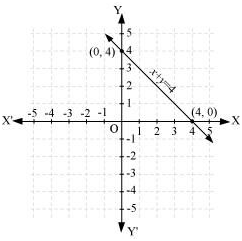(ii)It can be observed that x = 4, y = 2and x = 2, y = 0 are solutions of the aboveequation. Therefore, the solution table is as follows.

 x 4 2 y 2 0

The graph of the above equation is constructed as follows.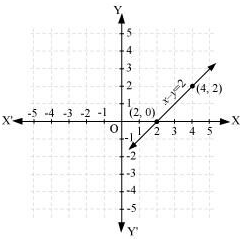(iii) y = 3x

It can be observed that x = −1, y =−3 and x = 1, y = 3 are solutions of theabove equation. Therefore, the solution table is as follows.

 x − 1 1 y − 3 3

The graph of the above equation is constructed as follows.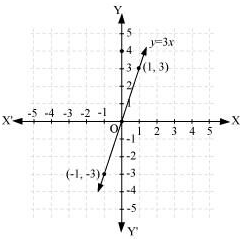(iv) 3 = 2x + y

It can be observed that x = 0, y = 3and x = 1, y = 1 are solutions of the aboveequation. Therefore, the solution table is as follows.

 x 0 1 y 3 1

The graph of this equation is constructed as follows.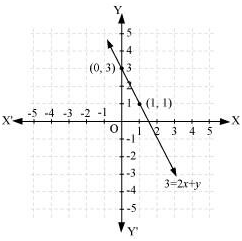Question 2 :

Give the equations of two lines passing through (2, 14). How many moresuch lines are there, and why?

It can be observed that point (2, 14) satisfies the equation 7x − y =0 and

x − y +12 = 0.

Therefore, 7x − y = 0 and x − y +12 = 0 are two lines passing through point (2, 14).

As it is known that through one point, infinitenumber of lines can pass through, therefore, there are infinite lines of suchtype passing through the given point.

Question 3 :

If the point (3, 4) lies on the graph of the equation 3y = ax +7, find the value of a

Putting = 3 and y = 4 in the givenequation,

3y = ax + 7

3 (4) = (3) + 7

5 = 3a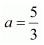Question 4 : The taxi fare in a city is as follows: For the first kilometre, the fares is Rs 8 and for the subsequent distance it is Rs 5 per km. Taking the distance covered as x km and total fare as Rs y, write a linear equation for this information, and draw its graph.

Total distance covered = x km

Fare for 1st kilometre = Rs 8

Fare for the rest of the distance = Rs (x − 1) 5

Total fare = Rs [8 + (− 1) 5]

= 8 + 5x −5

= 5x + 3

5x − + 3 = 0

It can be observed that point (0, 3) and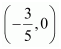satisfies the above equation. Therefore, these are the solutions ofthis equation.

 x 0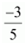y 3 0

The graph of this equation is constructed as follows.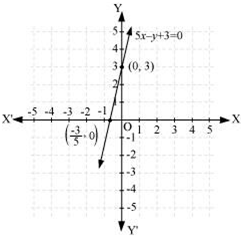Here,it can be seen that variable x and y arerepresenting the distance covered and the fare paid for that distancerespectively and these quantities may not be negative. Hence, only those valuesof x and y which are lying in the 1st quadrantwill be considered.

Question 5 :

From the choices given below, choose the equation whose graphs aregiven in the given figures.

 For the first figure For the second figure (i) y = x (i) y = x +2 (ii) x + y = 0 (ii) y = x − 2 (iii) y = 2x (iii) y = − x + 2 (iv) 2 + 3y = 7x (iv) x + 2y = 6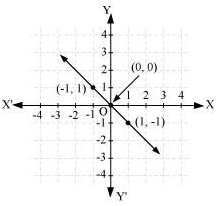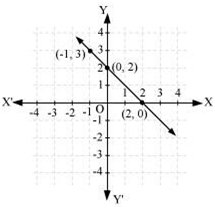Points on the given line are (−1, 1), (0, 0), and (1, −1).

It can be observed that the coordinates of the points of the graphsatisfy the equation x + y = 0.Therefore, x + y = 0 is the equationcorresponding to the graph as shown in the first figure.

Hence, (ii) is the correct answer.Points on the given line are (−1, 3), (0, 2), and (2, 0). It can beobserved that the coordinates of the points of the graph satisfy the equation y =− x + 2.

Therefore, = − x + 2 is theequation corresponding to the graph shown in the second figure.

Hence, (iii) is the correct answer.

Question 6 :

If the work done by a body on application of a constant force isdirectly proportional to the distance travelled by the body, express this inthe form of an equation in two variables and draw the graph of the same bytaking the constant force as 5 units. Also read from the graph the work donewhen the distance travelled by the body is

(i) 2 units (ii) 0 units

Let the distance travelled and the work done by the body be x and y respectively.

Work done distance travelled

y  x

y = kx

Where, k is a constant

If constant force is 5 units, then work done y = 5x

It can be observed that point (1, 5) and (−1,−5) satisfy the above equation. Therefore, these are the solutions of thisequation. The graph of this equation is constructed as follows.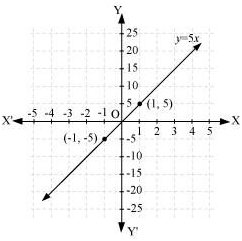(i)From the graphs, it can be observed that the value of correspondingto x = 2 is 10. This implies that the work done by the body is10 units when the distance travelled by it is 2 units.

(ii) From the graphs, it can be observed that the value of correspondingto x = 0 is 0. This implies that the work done by the body is0 units when the distance travelled by it is 0 unit.

Question 7 :

Yamini and Fatima, two students of Class IX of a school, togethercontributed Rs 100 towards the Prime Minister’s Relief Fund to help theearthquake victims. Write a linear equation which satisfies this data. (You maytake their contributions as Rs x and Rs y.) Drawthe graph of the same.

Let the amount that Yamini and Fatima contributed be x and y respectivelytowards the Prime Minister’s Relief fund.

Amount contributed by Yamini + Amount contributed by Fatima = 100

y =100

It can be observed that (100, 0) and (0, 100) satisfy the aboveequation. Therefore, these are the solutions of the above equation. The graphis constructed as follows.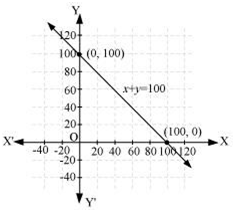Here, it can be seen that variable x and y arerepresenting the amount contributed by Yamini and Fatima respectively and thesequantities cannot be negative. Hence, only those values of x and y whichare lying in the 1st quadrant will be considered.

Question 8 :

In countries like USA and Canada, temperature is measured inFahrenheit, whereas in countries like India, it is measured in Celsius. Here isa linear equation that converts Fahrenheit to Celsius: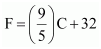(i) Draw the graph of the linear equation above using Celsius for x-axisand Fahrenheit for y-axis.

(ii) If the temperature is 30°C, what is the temperature in Fahrenheit?

(iii) If the temperature is 95°F, what is the temperature in Celsius?

(iv) If the temperature is 0°C, what is the temperature in Fahrenheitand if the temperature is 0°F, what is the temperature in Celsius?

(v) Is there a temperature which is numerically the same in bothFahrenheit and Celsius? If yes, find it.

Answer 8 : (i)It can be observed that points (0, 32) and (−40, −40) satisfy the givenequation. Therefore, these points are the solutions of this equation.

The graph of the above equation is constructed as follows.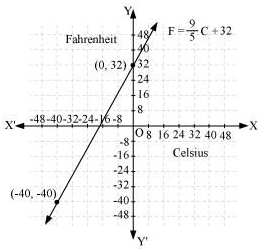(ii) Temperature = 30°C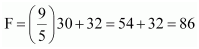Therefore, the temperature in Fahrenheit is 86°F.

(iii) Temperature = 95°F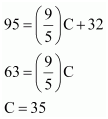Therefore, the temperature in Celsius is 35°C.

(iv)If C = 0°C, then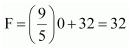Therefore, if C = 0°C, then F = 32°F

If F = 0°F, then